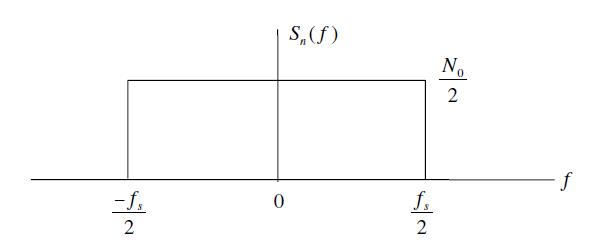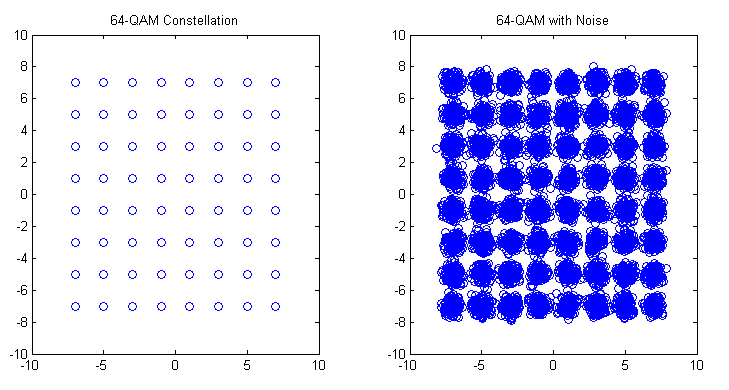# Wireless Channel Modeling: Back to Fundamentals

When a wireless signal travels from a transmitter (Tx) to a receiver (Rx) it undergoes some changes. In simple terms the signal s(t) is scaled by a factor h(t) and noise n(t) is added at the receiver. Let’s take this discussion forward with a simple example. Suppose the Tx transmits one of two possible symbols, +1 or -1. In technical lingo this is called Binary Phase Shift Keying (BPSK). If the channel scaling factor is 0.1 we will either get a +0.1 or -0.1 at the Rx to which AWGN noise is added. The noise is random in nature (having a Gaussian distribution) but for simplicity we assume that it can have one of two values, +0.01 or -0.01.

Continue reading Wireless Channel Modeling: Back to Fundamentals

# Noise Calibration in Simulation of Communication Systems

We have been using a wireless signal model in our simulations without going into the details of noise calibration for simulation. In this article we discuss this. Lets assume the received signal is given as

r(t)=s(t)+n(t)

where r(t) is the received signal s(t) is the transmitted signal and n(t) is the Additive White Gaussian Noise (AWGN). Channel fading is ignored at the moment. Signal to noise ratio for simulation of digital communication systems is given as

ρ=Eb/No (1)

Where Eb is the energy per bit and No is the noise Power Spectral Density (PSD). We also know that for the case of Additive White Gaussian Noise the noise power is given as [Tranter]

σ^2=(No/2)*fs

No=2*(σ^2)/fsWhere σ is the standard deviation of noise and fs is the sampling frequency.  Substituting in equation 1 we get

ρ=Eb/No=Eb/(2*σ^2/fs )

Eb/No=(Eb*fs)/(2*σ^2)

σ^2=(Eb*fs)/(2*Eb/No )

σ=√((Eb*fs)/(2*Eb/No ))

If the energy per bit and the sampling frequency is set to 1 the above equation reduces to

σ=√(1/(2*Eb/No ))

The simulation software can thus calculate the noise standard deviation (or variance) for each value of Eb/No in the simulation cycle. The following piece of MATLAB code generates AWGN with the required power and adds it to the transmitted signal.

```s=sign(rand-0.5);      % Generate a symbol
sigma=1/sqrt(2*EbNo);  % Calculate noise standard deviation
n=sigma*randn;         % Generate AWGN with the required std dev
r=s+n;                 % Add noise to the signal```

How can we assume that energy per bit and sampling frequency is equal to one and are we breaking some discrete time signal processing rule here. This will be discussed in a later post.

# M-QAM Bit Error Rate in AWGN

Quadrature Amplitude Modulation has been adopted by most wireless communication standards such as WiMAX and LTE. It provides higher bit rates and consequently higher spectral efficiencies. It is usually used in conjunction with Orthogonal Frequency Division Multiplexing (OFDM) which provides a simple technique to overcome the time varying frequency selective channel.

We have previously discussed the formula for calculating the bit error rate (BER) of QAM in AWGN. We now calculate the same using a simple Monte Carlo Simulation.

```%%%%%%%%%%%%%%%%%%%%%%%%%%%%%%%%%%%%%%%%%%%%%%%%%%%%%%%%%%%%%%%%%%
% FUNCTION THAT CALCULATES THE BER OF M-QAM IN AWGN
% n_bits: Input, number of bits
% M: Input, constellation size
% EbNodB: Input, energy per bit to noise power spectral density
% ber: Output, bit error rate
% Copyright RAYmaps (www.raymaps.com)
%%%%%%%%%%%%%%%%%%%%%%%%%%%%%%%%%%%%%%%%%%%%%%%%%%%%%%%%%%%%%%%%%%

function[ber]= QAM_AWGN(n_bits, M, EbNodB)

% Transmitter
k=log2(M);
EbNo=10^(EbNodB/10);
x=transpose(round(rand(1,n_bits)));
h1=modem.qammod(M);
h1.inputtype='bit';
h1.symbolorder='gray';
y=modulate(h1,x);

% Channel
Eb=mean((abs(y)).^2)/k;
sigma=sqrt(Eb/(2*EbNo));
w=sigma*(randn(1,n_bits/k)+1i*randn(1,n_bits/k));
r=y+w';

h2=modem.qamdemod(M);
h2.outputtype='bit';
h2.symbolorder='gray';
h2.decisiontype='hard decision';
z=demodulate(h2,r);
ber=(n_bits-sum(x==z))/n_bits
return
%%%%%%%%%%%%%%%%%%%%%%%%%%%%%%%%%%%%%%%%%%%%%%%%%%%%%%%%%%%%%%%%%%
```The above function basically has three inputs and one output. The inputs are the number of bits to be passed through the channel, the size of the constellation and the signal to noise ratio in dB. The output is the bit error rate (BER). The simulation can be divided into three section namely the transmitter, the channel and the receiver. In this simulation we have used Gray coding which gives us about a dB of improvement at low to medium signal to noise ratio.

As seen above the BER obtained through our simulation matches quite well with the BER obtained through the theoretical formula. Each additional bit per symbol required about 2dB extra in signal to noise ratio to achieve the same bit error rate.

Lastly we explain some of the terms used above.

AWGN

All wireless receivers suffer from thermal noise which is a function of absolute temperature and bandwidth of the receiver. This noise is added to the received signal and makes detection of weak signals a major challenge. Just to given you an idea typical GSM receivers have a noise floor of -113 dBm. Therefore, if the received signal has a power of -100 dBm we get a signal to noise ratio (SNR) of 13 dB. In simulation this noise is usually modeled as a Gaussian Random Process. It is additive, as opposed to channel impairments which are multiplicative and has a flat spectrum (thus called White Noise).

Gray Coding

When using QAM modulation, each QAM symbol represents 2,3,4 or higher number of bits. That means that when a symbol error occurs a number of bits are reversed. Now a good way to do the bit-to-symbol assignment is to do it in a way such that no neighboring symbols differ by more than one bit e.g. in 16-QAM, a symbol that represents a binary word 1101 is surrounded by four symbols representing 0101, 1100, 1001 and 1111. So if a symbol error is made, only one bit would be in error. However, one must note that this is true only in good signal conditions. When the SNR is low (noise has a higher magnitude) the symbol might be displaced to a location that is not adjacent and we might get higher number of bits in error.

Hard Decision

The concept of hard decision decoding is important when talking about channel coding, which we have not used in the above simulation. However, we will briefly explain it here. Hard decision is based on what is called “Hamming Distance” whereas soft decision is based on what it called “Euclidean Distance”. Hamming Distance is the distance of a code word in binary form, such as 011 differs from 010 and 001 by 1. Whereas the Euclidean distance is the distance before a decision is made that a bit is zero or one.  So if the received sequence is 0.1 0.6 0.7 we get a Euclidean distance of 0.8124 from 010 and 0.6782 from 001. So we cannot make a hard decision about which sequence was transmitted based on the received sequence of 011. But based on the soft metrics we can make a decision that 001 was the most likely sequence that was transmitted (assuming that 010 and 001 were the only possible transmitted sequences).

# Detecting Sinusoids in Noise

We have previously discussed the problem of detecting two closely spaced sinusoids using the Discrete Fourier Transform (DFT). We assumed that the data set we got was pure i.e. there was no noise. However, in reality this is seldom the case. There is always some noise, corrupting the signal. Let us now see how it effects the detection problem.

We consider Additive White Gaussian Noise (AWGN) as the corrupting source. The noise power is set equal to the power of the two sinusoids i.e. we have an SNR of 0 dB. This is quite a severe case, the noise power is usually a few dB below the signal power. We are also bounded by the number of samples, N=64, giving us a resolution of 15.87 Hz.

```clear all
close all

fm1=100;
fm2=120;
fs=1000;
Ts=1/fs;
N=64;
t=0:Ts:(N-1)*Ts;

x1=sqrt(2)*cos(2*pi*fm1*t);
x2=sqrt(2)*cos(2*pi*fm2*t);
wn=randn(1,N);
x=x1+x2+wn;
W=exp(-j*2*pi/N);

n=0:N-1;
k=0:N-1;

X=x*(W.^(n'*k));

plot(k/N,20*log10(abs(X)))
xlabel ('Normalized Frequency')
ylabel ('X(dB)')```

The data is plotted on a logarithmic scale so that we can compare the signal and noise  power levels.

It is observed that we still have two peaks around the required frequency bins but there are also a number of false peaks. These peaks are around 10 dB lower than the signal peaks and should not cause a false detection. So the signal to noise ratio of 0 dB in the time domain is translated to a signal to noise ratio of about 10 dB in the frequency domain (this can be realized using an appropriate filter).

# QAM Theoretical BER in AWGN

Quadrature Amplitude Modulation (QAM) is an important modulation scheme as it allows for higher data rates and spectral efficiencies. The bit error rate (BER) of QAM can be calculated through Monte Carlo simulations. However this becomes quite complex as the constellation size of the modulation schemes increases. Therefore a theoretical approach is sometimes preferred. The BER for Gray coded QAM, for even number of bits per symbol, is shown below.

Gray coding ensures that a symbol error results in a single bit error. The code for calculating the theoretical QAM BER for k even (even number of bits per symbol) is given below. The formula for calculating the BER for k odd is different, however, the formula given below can be used a first estimate.

```EbNodB=-6:2:24
EbNo=10.^(EbNodB/10);
k=8;
M=2^k;
x=sqrt(3*k*EbNo/(M-1));
Pb=(4/k)*(1-1/sqrt(M))*(1/2)*erfc(x/sqrt(2));
semilogy(EbNodB,Pb)```

Note:
1. Each additional bit/symbol requires about 2dB extra in SNR to achieve the same BER.
2. 4-QAM is essentially QPSK modulation.